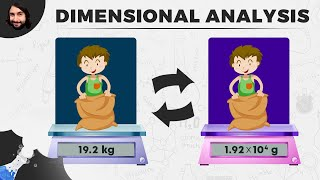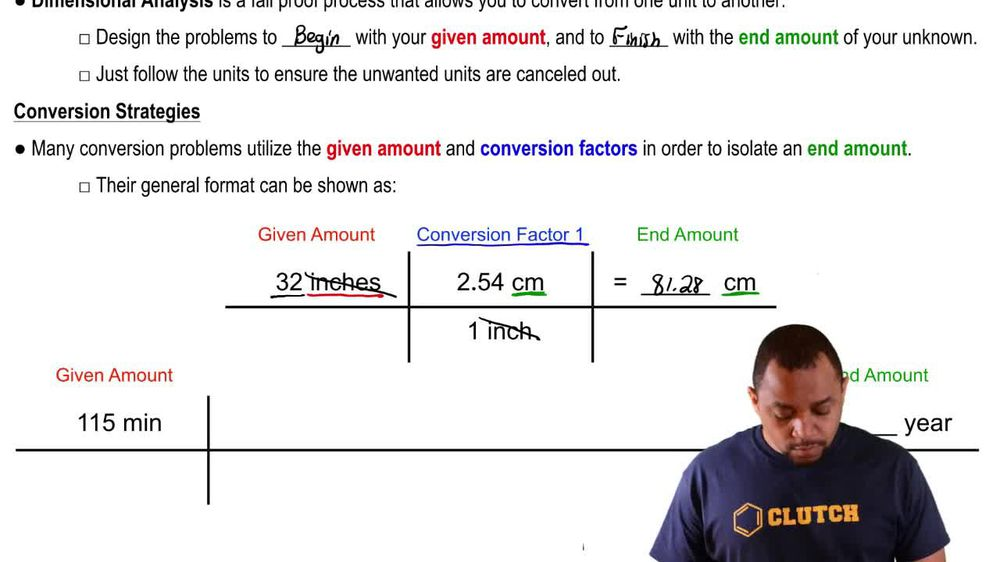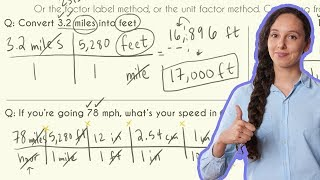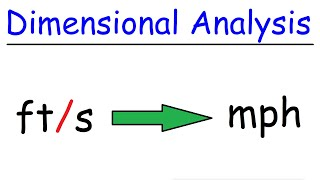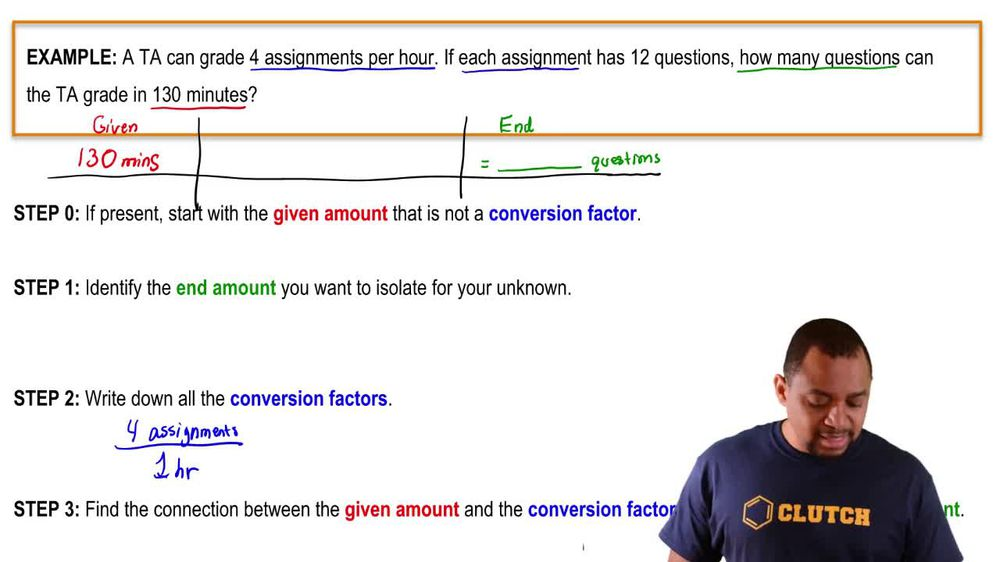Start typing, then use the up and down arrows to select an option from the list.
1. 1. Intro to General Chemistry2. Dimensional Analysis
Problem

# Perform each unit conversion. a. 1.4 in to mm b. 116 ft to cm c. 1845 kg to lb d. 815 yd to km

Relevant Solution3m
Play a video:
Hello everyone in this video. I'm gonna go ahead and convert this unit into this unit. So if you guys are not ready, this capital a with little bubble on top that stands for the unit Angstrom. So what is the conversion from Angstrom two. This is not something that I know at least I'm going to go ahead and kind of break this down and let me write out my game plan. I like to read this out so I can make sure that I will eventually get to my desire unit from dimensional analysis. So I'm going to start off with my angstrom because that is what we are given. So I have no other choice and going from Ekstrom, I know the conversion to meters and from meters into millimeters. Again, there's something that I know so sometimes you can do even more steps or you do maybe know the direct conversion. So you go ahead and use less steps. But this is just my game plan. Alright, so some information that we do know. So the convergence, we know that one Angstrom, There's going to be 10 to the native 10 power meters. We also know that for everyone milla meter, There's going to be 10 to the negative 3rd m. So these are crucial bits of information that we know and we can use for our conversions for the dimension analysis. We scroll down just a little bit so we have some space here. So we're going to be starting off with our angstrom and we are given several points, five, three and streams and I'm going to use this conversion right here. So I'm gonna go ahead and cancel out the Angstrom and go two m. So to cancel an angstrom, I have to go ahead and put that same unit on the bottom and we're going to meters. So using this right here, I know that everyone angstrom is going to be 10 to the negative m and going from meters into millimeters. I'm going to have to consult the meters. So meters on the bottom to consult with the top and milla meters using this piece of information. So using that, I know that every one millimeter We'll have 10 to the negative three m and like I said, angstrom will cancel and meters will cancel leaving us with just the millimeters that we desire over here. Alright, so putting all of this into my calculator, I will get a value of 5.3 times 10 to the -8 mila meters. And that is going to be my final answer for this dimension analysis problem